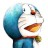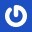# 杰拉斯的博客

## [ACM_ZOJ_1003]Crashing Balloon杰拉斯 | 时间：2012-05-22, Tue | 22,313 views

### Crashing Balloon

Time Limit: 2 Seconds Memory Limit: 65536 KB

#### Description

On every June 1st, the Children's Day, there will be a game named "crashing balloon" on TV. The rule is very simple. On the ground there are 100 labeled balloons, with the numbers 1 to 100. After the referee shouts "Let's go!" the two players, who each starts with a score of "1", race to crash the balloons by their feet and, at the same time, multiply their scores by the numbers written on the balloons they crash. After a minute, the little audiences are allowed to take the remaining balloons away, and each contestant reports his\her score, the product of the numbers on the balloons he\she's crashed. The unofficial winner is the player who announced the highest score.

Inevitably, though, disputes arise, and so the official winner is not determined until the disputes are resolved. The player who claims the lower score is entitled to challenge his\her opponent's score. The player with the lower score is presumed to have told the truth, because if he\she were to lie about his\her score, he\she would surely come up with a bigger better lie. The challenge is upheld if the player with the higher score has a score that cannot be achieved with balloons not crashed by the challenging player. So, if the challenge is successful, the player claiming the lower score wins.

So, for example, if one player claims 343 points and the other claims 49, then clearly the first player is lying; the only way to score 343 is by crashing balloons labeled 7 and 49, and the only way to score 49 is by crashing a balloon labeled 49. Since each of two scores requires crashing the balloon labeled 49, the one claiming 343 points is presumed to be lying.

On the other hand, if one player claims 162 points and the other claims 81, it is possible for both to be telling the truth (e.g. one crashes balloons 2, 3 and 27, while the other crashes balloon 81), so the challenge would not be upheld.

By the way, if the challenger made a mistake on calculating his/her score, then the challenge would not be upheld. For example, if one player claims 10001 points and the other claims 10003, then clearly none of them are telling the truth. In this case, the challenge would not be upheld.

Unfortunately, anyone who is willing to referee a game of crashing balloon is likely to get over-excited in the hot atmosphere that he\she could not reasonably be expected to perform the intricate calculations that refereeing requires. Hence the need for you, sober programmer, to provide a software solution.

#### Input

Pairs of unequal, positive numbers, with each pair on a single line, that are claimed scores from a game of crashing balloon.

#### Output

Numbers, one to a line, that are the winning scores, assuming that the player with the lower score always challenges the outcome.

#### Sample Input

```343 49
3599 610
62 36
```

```49
610
62
```

#### Source

ZOJ1003

```#include <iostream>
#define MAX 100
using namespace std;

bool UsedBalloon[MAX+1];	//因子是否已经被分解过

bool CanFactor(int num, int i = 1){	//能否分解且因子与UsedBalloon不冲突
if(i > MAX)	//寻找的因子超出MAX
return false;
if(num == 1){	//分解成功
return true;
}else{
while(i <= MAX)	//找到一个可以整除并不大于MAX的因子
{
if(UsedBalloon[i] == false && num % i == 0){
break;
}
else{
i++;
}
}
if(i > MAX)
return false;
if(CanFactor(num / i, i + 1))	//num / i能分解
return true;
else
return CanFactor(num, i + 1);	//继续找num的下一个因子
}
}

bool IsOK(int numA, int numB, int i = 1){
if(i > MAX)
return false;
if(numA == 1)	//A分解完，难点在这里解决，每一种A的分解方案都寻找B是否存在与A不冲突的分解方案
return CanFactor(numB);	//看是否B能够被分解且因子与当前A分解因子不冲突
while(i <= MAX)
{
if(numA % i == 0)
{
break;
}
else
{
i++;
}
}
if(i > MAX)
return false;
UsedBalloon[i] = true;	//分解出该因子
if(IsOK(numA / i, numB, i + 1))	//numA / i能分解
return true;
UsedBalloon[i] = false;	//该因子不分解
return IsOK(numA, numB, i + 1);	//继续找numA的下一个因子
}

int GetWinner(int numA, int numB){
for(int i = 1; i < MAX + 1;i++){
UsedBalloon[i] = false;
}
if(numA < numB)
swap(numA, numB);	//确保B是挑战者
if(!CanFactor(numB) && numB > MAX)	//如果B不能分解且B大于100
return numA;	//说明B是撒谎的，A赢
else if(IsOK(numA, numB)){	//如果A, B均可分解且因子不同
return numA;
}else{
return numB;
}
}

int main()
{
int numA, numB;
while(cin >> numA >> numB){
cout << GetWinner(numA, numB) << endl;
}
return 0;
}
```

```#include<stdio.h>
int flag1, flag2;	//分别表示n, m是否已被成功分解
void dfs(int n, int m, int fac = 100){
if(flag1)	//如果n, m均可分解且因子不同
return;
if(n == 1 && m == 1){	//n, m均分解完
flag1 = 1;
flag2 = 1;
return;
}
if(m == 1){ //m分解完
flag2 = 1;
}
if (fac < 2)
return;
if(n % fac == 0)	//精华之处，对同一个因子不同时分解n, m，难点解决
dfs(n / fac, m, fac - 1);
if(m % fac == 0)
dfs(n, m / fac, fac - 1);
dfs(n, m, fac - 1);
}

int main(){
int n, m;
while(~scanf("%d%d", &n, &m)){
if(m > n){ //交换m, n
n = m ^ n;
m = m ^ n;
n = m ^ n;
}
flag1 = 0;
flag2 = 0;
dfs(n, m);
if(flag1 || !flag2)	//如果n成功分解货m无法成功分解
printf("%d\n", n);
else
printf("%d\n", m);
}
return 0;
}
```

#### 仅有一条评论 »

1.ACM之家

太棒了，找的就是这一题。转走了